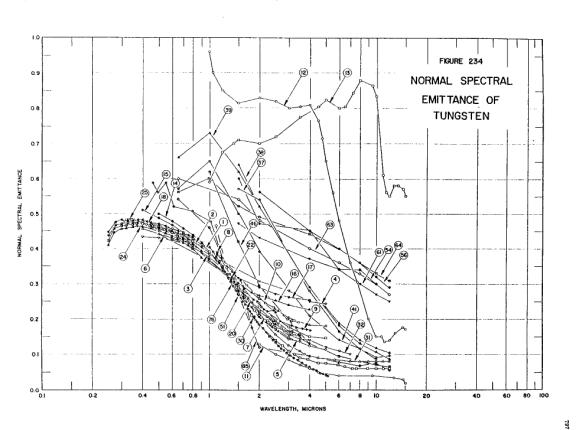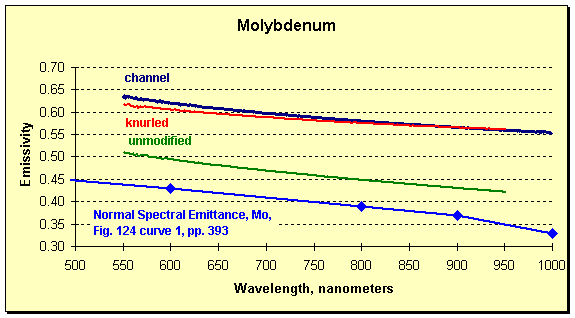# Metals

The emissivity of metals often changes with wavelength. This means it is very difficult to determine what emissivity should be entered in passive, traditional pyrometers (all those that do not determine the emissivity during measurement). An example or two will show the difficulty.Emissivity of tungsten is particularly well-known because of its application to lighting.Spectral emissivity of molybdenum; the only difference between traces 1, 2, and 3 is the temperature of the target.

Both graphs are taken from Thermophysical Properties of Matter, Vol. 7: Thermal Radiative Properties, eds. Y.S. Touloukian and D.P. DeWitt, IFI/Plenum, New York, 1970.

The problems are obvious. The parameter needed to determine the temperature changes with temperature. The change with wavelength means the wavelength response of the pyrometer must be known to proceed.

To calculate the emissivity (or relative emissivity) for a particular pyrometer using information such as that above the following must be true:

• the conditions of the measurement must match an exact set of conditions reported;
• the conditions must not change during the measurement;
• the instrument’s response as a function of wavelength must be known.

Then the correct way to proceed would be to integrate the product of instrument bandwidth and the appropriate emissivity as a function of wavelength (must be done for both bandwidths for a ratio instrument), and use the resulting weighted emissivity (or emissivities).

The probability that all the required information is at hand is small. Even if it were, the change with temperature will always introduce uncertainty in both the temperature measurement and the mind of the user.

The FAR SpectroPyrometer removes the need for such gyrations. A single measurement generated each of the curves in the following figures.

A tungsten filament lamp was the temperature source for the following curve. The FAR data was normalized to the CRC Handbook data because the filament did not fill the field of view.Tungsten results from CRC Handbook of Chemistry and Physics, 60th Edition, pp. E-381, ed. R.C. Weast, CRC Press, Boca Raton, 1979; FAR data normalized to CRC at 650 nm.

A molybdenum target was modified in two ways: first, by milling a small channel along part of its length, and second, by lightly knurling the surface. These were done to enhance emissivity for purposes of radiative heat transfer. A SpectroPyrometer scan now compared the two modifications with the unmodified metal.The curve identified as Normal Spectral Emittance is taken from the Thermophysical Properties of Matter, cited above. The other curves result from SpectroPyrometer measurements on a molybdenum sample modified as described.

The agreement between the published data and the FAR results is quite good. But it is one thing to add another trace to the multitude of curves on the published data and note that it fits, and quite another to try to pick one to use for a temperature measurement.